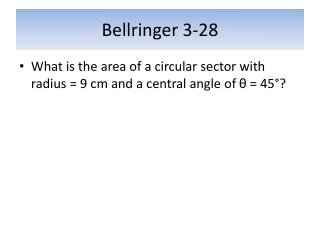DownloadDownload PresentationBellringer 3-28

# Bellringer 3-28

Télécharger la présentation## Bellringer 3-28

- - - - - - - - - - - - - - - - - - - - - - - - - - - E N D - - - - - - - - - - - - - - - - - - - - - - - - - - -
##### Presentation Transcript

1. Bellringer 3-28 • What is the area of a circular sector with radius = 9 cm and a central angle of θ = 45°?

2. Homework Section 5.2 • Pg. 251 (17, 21, 24, 28, 29) • 17. 20𝜋/3 (20.9 cm) • 21. 5𝜋/36 (.44 in) • 24. 35.8° • 28. 65.45 ft2 • 29. 16.97 in2

3. Section 5.3 – Circular Functions • Definition of Sine and Cosine • If the terminal side of an angle θ in standard position intersects the unit circle at P(x,y), then cos θ = x andsin θ = y.

4. Sine and Cosine • Ex. What is the sin 90°? (Draw circle and find coordinates.)

5. Sine and Cosine (2) • What is the cos 𝜋?

6. Sine and Cosine (Functions of an Angle) • For any angle in standard position with θ, a point P(x,y) on its terminal side, and r = , the sine and cosine functions of θ are as follows: sin θ = cos θ =

7. Example 1 • Find the values of sine and cosine functions of an angle in standard position with measure θ if the point with coordinates (3,4) lies on its terminal side.

8. Example 2 • Find sin θ when cos θ = and the terminal side of θ is in the first quadrant.

9. 6 Trigonometric Functions • Sine, Cosine, Tangent, Cosecant, Secant, Cotangent • sin θ = cos θ = tan θ = • csc θ = sec θ = cot θ = • Notice cosecant is the reciprocal of sine… • csc θ = 1/sin θ

10. Example • The terminal side of an angle θ in standard position contains the point with coordinates (8, -15). Find the values of all 6 trig. Functions.

11. Chart for signs in Quadrants

12. Homework 5.3 • Pg. 260 (25, 28, 32)